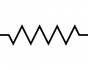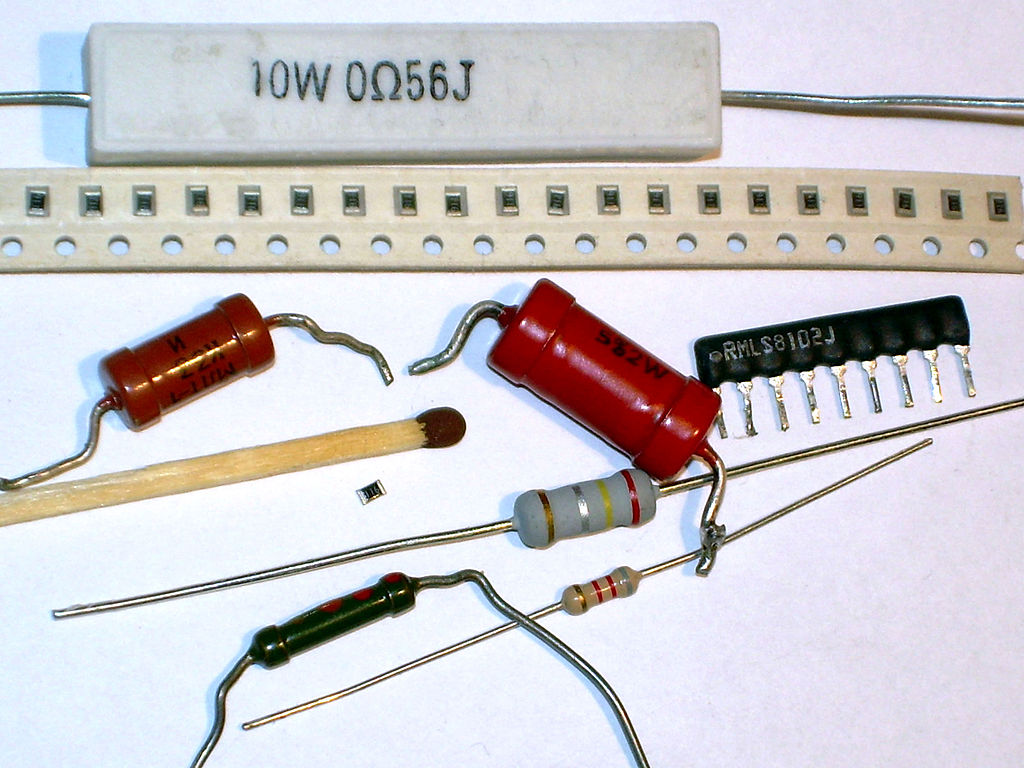### 8. Resistors

A resistor is an electronic component that limits the flow of electrons. In doing so, it dissipates energy in the form of heat.The amount of resistance that a resistor offers is measured in Ohms. The symbol for ohms is the Greek omega symbol - Ω.

In terms of electronics, the resistor reduces electrical current by a precise amount. If you consider that in a circuit you typically have a fixed input voltage, and resistors offer a fixed amount of resistance, you can then use Ohm's Law to determine how much a resistor will limit current. This is useful in a number of scenarios, including working with LEDs.To measure ohms with a multimeter, turn the dial to the Ω symbol and selecting the proper range.

##### Resistor values
Determining how much resistance a resistor offers is a little trickier and can be established by deciphering the coloured stripes from left to right. You will typically see four stripes, but you may also encounter resistors with five.When reading a resistor with four stripes, the first two stripes are combined together to form a number between 1 and 99. The third marking is the multiplier. The last marking determines the tolerance.A resistor with a gold band has a resistance with a +/- 5% tolerance or - you could say - margin of error. What this means is that the resistance can be over or under its value by 5%. So, if you had a 100K resistor and measured with a multimeter, it could read anywhere from 95K to 105K. This will be alright for the purposes of this course.

##### Variable Resistors

A variable resistor is a resistor whose value varies within a set range.The simplest and most common variable resistor is the potentiometer. Every time you use a slider or knob on an electrical device, you are using a potentiometer. For instance, every single mechanical light dimmer you have ever used is a potentiometer.Potentiometers come in a wide range of resistances, and have a physical actuator that sweeps from 0 ohms resistance to whatever value is marked upon it.

By connecting a wire to either one of its outer terminals, and another to the centre terminal, we can wire a potentiometer as a variable resistor.

We can always check which pin is which with a multimeter.